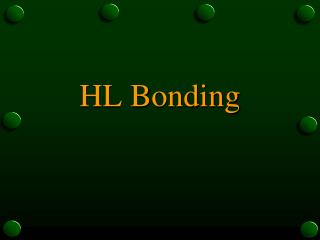# HL Bonding - PowerPoint PPT PresentationDownload PresentationHL Bonding

HL BondingDownload Presentation## HL Bonding

- - - - - - - - - - - - - - - - - - - - - - - - - - - E N D - - - - - - - - - - - - - - - - - - - - - - - - - - -
##### Presentation Transcript

1. HL Bonding

2. HL Bonding Sigma & pi bonds

3.  and  bonds • This goes beyond the Lewis representation

4.  and  bonds • This goes beyond the Lewis representation • Considers the combining of atomic orbitals to form molecular orbitals

5.  and  bonds • This goes beyond the Lewis representation • Considers the combining of atomic orbitals to form molecular orbitals • Remember - s orbitals are what shape?

6.  and  bonds • This goes beyond the Lewis representation • Considers the combining of atomic orbitals to form molecular orbitals • Remember - s orbitals are what shape? Spherical

7.  and  bonds • This goes beyond the Lewis representation • Considers the combining of atomic orbitals to form molecular orbitals • Remember - s orbitals are what shape? Spherical p orbitals are what shape?

8.  and  bonds • This goes beyond the Lewis representation • Considers the combining of atomic orbitals to form molecular orbitals • Remember - s orbitals are what shape? Spherical p orbitals are what shape? Peanut-shaped

9.  and  bonds • This goes beyond the Lewis representation • Considers the combining of atomic orbitals to form molecular orbitals • Remember - s orbitals are what shape? Spherical p orbitals are what shape? Peanut-shaped d orbitals are what shape?

10.  and  bonds • This goes beyond the Lewis representation • Considers the combining of atomic orbitals to form molecular orbitals • Remember - s orbitals are what shape? Spherical p orbitals are what shape? Peanut-shaped d orbitals are what shape? Doughnut-shaped

11.  and  bonds • This goes beyond the Lewis representation • Considers the combining of atomic orbitals to form molecular orbitals • Remember - s orbitals are what shape? Spherical p orbitals are what shape? Peanut-shaped d orbitals are what shape? Doughnut-shaped We will only consider s & p orbitals here

12. s orbitals are spherical p orbitals are dumbbell (or peanut) shaped From: http://www.grenvillescience.com/chemistry/y6l/asdef.html

13. Sigma () bonds • A bond situated symmetrically between 2 atoms

14. Sigma () bonds • A bond situated symmetrically between 2 atoms • Formed when 2 orbitals overlap along a line drawn between the 2 nuclei

15. Sigma () bonds • A bond situated symmetrically between 2 atoms • Formed when 2 orbitals overlap along a line drawn between the 2 nuclei • Occurs when 2 s orbitals overlap

16. Sigma () bonds • A bond situated symmetrically between 2 atoms • Formed when 2 orbitals overlap along a line drawn between the 2 nuclei • Occurs when 2 s orbitals overlap • e.g. H2 From: http://www.grenvillescience.com/chemistry/y6l/asdef.html

17. Occurs when an s orbital overlaps with a p orbital • E.g. HCl From: http://www.webchem.net/notes/chemical_bonding/orbitals_and_covalent_bonds.htm

18. Occurs when an s orbital overlaps with a p orbital • E.g. HCl • Occurs when two p orbitals overlap “head on” • E.g. Cl2, methane - CH4 From: http://www.webchem.net/notes/chemical_bonding/orbitals_and_covalent_bonds.htm

19. Pi () bond • Formed by the “sideways” overlap of two p orbitals • The overlap occurs both above and below the line drawn through the nuclei • E.g. ethene C2H4 From: http://www.everyscience.com/Chemistry/Physical/Theories_of_Bonding/.images/MolStcPiBond.gif

20. From: http://www.cofc.edu/~deavorj/102/images/organic/pi-bonds.jpg

21. When & What Type? • A single bond is always a sigma bond as a pi bond cannot form until after a sigma bond has formed • A double bond consists of one sigma bond and one pi bond • A triple bond consists of one sigma bond and two pi bonds. • As the electrons in the pi bonds are further from the nucleus they are weaker than sigma bonds

22. e.g. ethene • From: http://ibchem.com/IB/ibnotes/full/bon_htm/14.2.htm • The carbon-carbon double bond contains 1  and 1  bond. The carbon-hydrogen single bonds are all  bonds. • The ethene molecule contains a total of one  bond and five  bonds

23. Identify the number of  and  bonds in the following molecules.Explain your answer. • Nitrogen Gas • From: “http://www.windows.ucar.edu/physical_science/chemistry/n2_molecule_sm.gif” cannot be displayed, because it contains errors. • A triple bond so one sigma and one pi bond

24. Identify the number of  and  bonds in the following molecules.Explain your answer. • Ethyne • From: http://www2.eou.edu/chemweb/molmodel/mmp9f.html

25. Identify the number of  and  bonds in the following molecules.Explain your answer. • Ethyne • From: http://www2.eou.edu/chemweb/molmodel/mmp9f.html • 2 pi bonds in triple bond • 3 sigma bonds - 1 in triple bond and 1 in each of the carbon-hydrogen single bonds

26. Identify the number of  and  bonds in the following molecules.Explain your answer. • Buta-1,3-diene • What is its formula? • CH2CHCHCH2 • From: http://www.scibio.unifi.it/chimorg/cap04.html

27. Identify the number of  and  bonds in the following molecules.Explain your answer. • Buta-1,3-diene • What is its formula? • CH2CHCHCH2 • From: http://www.scibio.unifi.it/chimorg/cap04.html • Two pi bonds - one in each double bond • Eight sigma bonds - one in each of the double bonds (2) and one in each of the carbon-hydrogen single bonds (6)